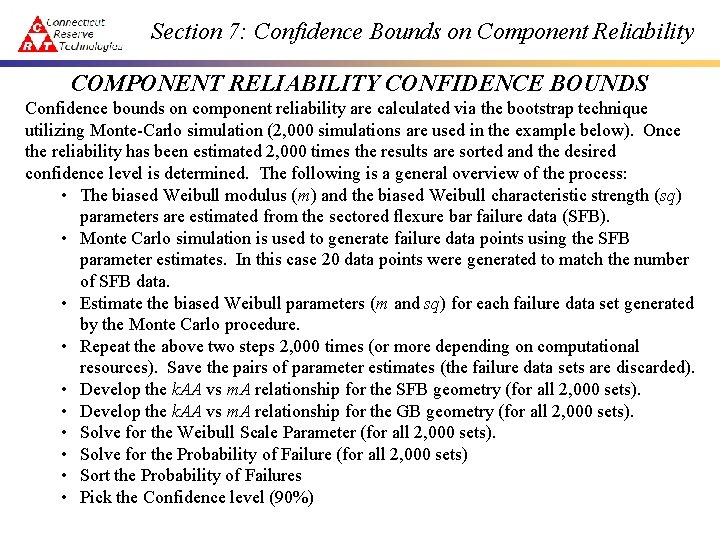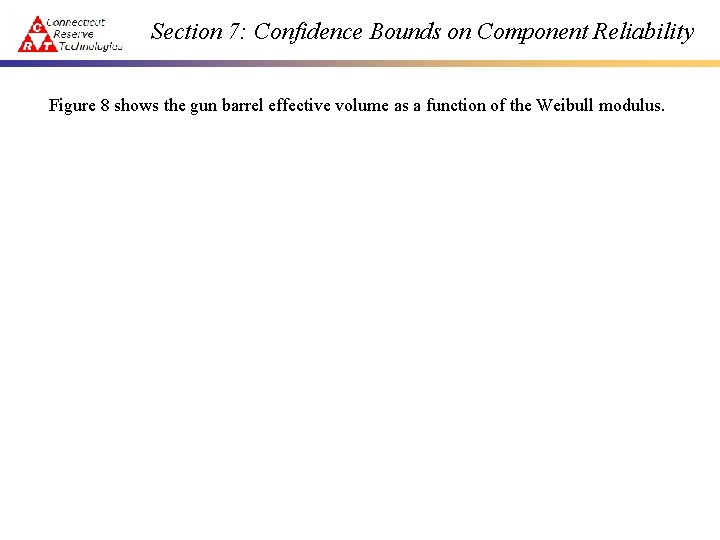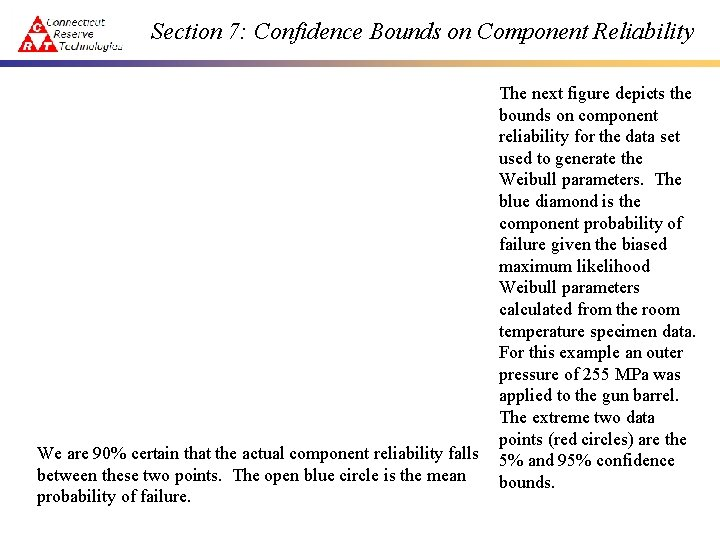# Section 7 Confidence Bounds on Component Reliability COMPONENT

• Slides: 11Section 7: Confidence Bounds on Component Reliability COMPONENT RELIABILITY CONFIDENCE BOUNDS Confidence bounds on component reliability are calculated via the bootstrap technique utilizing Monte-Carlo simulation (2, 000 simulations are used in the example below). Once the reliability has been estimated 2, 000 times the results are sorted and the desired confidence level is determined. The following is a general overview of the process: • The biased Weibull modulus (m) and the biased Weibull characteristic strength (sq) parameters are estimated from the sectored flexure bar failure data (SFB). • Monte Carlo simulation is used to generate failure data points using the SFB parameter estimates. In this case 20 data points were generated to match the number of SFB data. • Estimate the biased Weibull parameters (m and sq) for each failure data set generated by the Monte Carlo procedure. • Repeat the above two steps 2, 000 times (or more depending on computational resources). Save the pairs of parameter estimates (the failure data sets are discarded). • Develop the k. AA vs m. A relationship for the SFB geometry (for all 2, 000 sets). • Develop the k. AA vs m. A relationship for the GB geometry (for all 2, 000 sets). • Solve for the Weibull Scale Parameter (for all 2, 000 sets). • Solve for the Probability of Failure (for all 2, 000 sets) • Sort the Probability of Failures • Pick the Confidence level (90%)Section 7: Confidence Bounds on Component Reliability An initial failure data set must be available along with a finite element analysis for both the test specimen and the component. Flexure bar failure data was used for the failure set along with the finite element analysis of the sectored flexure bar. Here the fractographic analysis determined that all the specimens had failed due to surface related defects. The dimensions of the test specimen are sufficient if the specimen is a standard tensile specimen or a standard bend bar specimen. Otherwise, a finite element model of the test specimen is required. For this specific task the finite element model of the sectored flexure bar (SFB) created under an earlier contract was utilized. Relative to the SFB test specimen, the following is a list of specific steps associated with that particular specimen: a) Estimate the Weibull Parameters for the sectored flexure bar. For this specific task the SN 47 specimen data at 21ºC was used. Here the Weibull modulus is (m. A)SFB = 12. 01 and the Weibull characteristic strength is (sq. A)SFB = 642. 5Section 7: Confidence Bounds on Component Reliability b) Next a random number between 0 and 1 is generated, i. e. , c) Calculate a failure stress associated with the random number from (b) and the Weibull parameters from (a) using the expression d) Repeat (b and c) N times, where N is the number of sectored flexure data values in the original data set. For this particular data set N=20. 1. TABLE 2 – Generated Data Set i si 1 Eq Above 2 Eq Above … … N Eq AboveSection 7: Confidence Bounds on Component Reliability e) Repeat steps (b) through (e) as many times as is computational feasible (as noted earlier the effort being reported on here used 2, 000 repetitions). The simulated sectored flex bar Weibull modulus and characteristic strength, i. e. , the (m. A)SFB and (sq. A)SFB columns of Table 3 are thus generated. f) Estimate Weibull parameters for the data set generated in the previous steps using maximum likelihood estimators (MLE). g) Establish the relationship between the simulated sectored flex bar Weibull moduli and the effective area, i. e. , (m. A)SFB and (k. A)SFB. Use the maximum and minimum values of (m. A)SFB from Table 3 as the bounds on (m. A)SFB. Use the SFB finite element model to calculate (k. A)SFB as a function of (m. A)SFB for a few key values of (m. A)SFB, then use a linear fit for the remaining values. Complete the (k. A)SFB column in Table 3. In future work the linear fit would be refined with a spline. 1. TABLE 3 – Bootstrap J (m. A)SFB (sq. A)SFB (k. A)Barrel (s 0 A)Material Pf. Barrel 1 (m. A)1 ( sq A ) 1 (k. A)1 (s 0 A)1 Pf 1 2 (m. A)2 ( sq A ) 2 (k. A)2 (s 0 A)2 Pf 2 … … … … BS (m. A)BS (sq. A)BS (k. A)BS (s 0 A)BS Pf. BSSection 7: Confidence Bounds on Component Reliability i) Calculate the material characteristic strength or material scale parameter, (s 0 A)Material, as a function of (m. A)SFB, (sq. A)SFB, and (k. A)SFB using the following expression: j) Calculate the gun barrel probability of failure, Pf. Barrel, given m. A, s 0 A, (k. A)Barrel, and the smax for the gun barrel (from CARES) using the following expression: k) The use of this formula allows the Pf column in Table 3 to be populated without the repeated execution of CARES. This saves on computational resources. l) Sort by Pf in order to establish percentiles and then choose bounds based on the percentiles. Weib. Par calculates the confidence bounds on component reliability via integration with CARES. As Weib. Par stores and manipulates the data from Table 3 above, CARES is called to perform the probability of failure calculations on the finite element model.Section 7: Confidence Bounds on Component Reliability The following figures depict the results of the bootstrapping procedure outlined above. A finite element model of the SFB test specimen is shown below.Section 7: Confidence Bounds on Component Reliability The next figure shows a plot of effective area as a function of the Weibull modulus with values from both the previous contract work (as blue diamonds) along with those generated through the bootstrap routines (green asterisks). The linear fit between the key values is shown as the orange line.Section 7: Confidence Bounds on Component Reliability Figure 8 shows the gun barrel effective volume as a function of the Weibull modulus.Section 7: Confidence Bounds on Component Reliability We are 90% certain that the actual component reliability falls between these two points. The open blue circle is the mean probability of failure. The next figure depicts the bounds on component reliability for the data set used to generate the Weibull parameters. The blue diamond is the component probability of failure given the biased maximum likelihood Weibull parameters calculated from the room temperature specimen data. For this example an outer pressure of 255 MPa was applied to the gun barrel. The extreme two data points (red circles) are the 5% and 95% confidence bounds.Section 7: Confidence Bounds on Component Reliability For comparison sake in this hypothetical example we will assume that even though we are increasing number of specimens, the Weibull parameters remain the same. The question may be proposed, what effect does the limited information (only 20 failure stresses in this case) have on the bounds on reliability? To answer this question the bootstrap technique was rerun for a variety of specimen data set sizes. Please note that these are hypothetical results in the sense that if we really broke 20 more specimens to increase our specimen data set to 40 then it is unlikely that the recalculated Weibull parameters would be the same as for the original 20 data points.Section 7: Confidence Bounds on Component Reliability